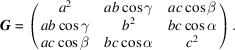International
Tables for
Crystallography
Volume A
Space-group symmetry
Edited by M. I. Aroyo

International Tables for Crystallography (2016). Vol. A, ch. 1.3, pp. 23-24

## Section 1.3.2.2. Metric properties

B. Souvigniera*

aRadboud University Nijmegen, Faculty of Science, Mathematics and Computing Science, Institute for Mathematics, Astrophysics and Particle Physics, Postbus 9010, 6500 GL Nijmegen, The Netherlands
Correspondence e-mail: souvi@math.ru.nl

#### 1.3.2.2. Metric properties

| top | pdf |

In the three-dimensional vector space, the norm or length of a vector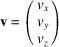is (due to Pythagoras' theorem) given by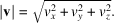From this, the scalar product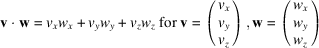is derived, which allows one to express angles by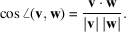The definition of a norm function for the vectors turnsinto a Euclidean space. A lattice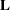that is contained ininherits the metric properties of this space. But for the lattice, these properties are most conveniently expressed with respect to a lattice basis. It is customary to choose basis vectors a, b, c which define a right-handed coordinate system, i.e. such that the matrix with columns a, b, c has a positive determinant.

#### Definition

For a lattice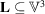with lattice basisthe metric tensor ofis the 3 × 3 matrix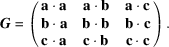Ifis the 3 × 3 matrix with the vectors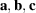as its columns, then the metric tensor is obtained as the matrix product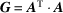. It follows immediately that the metric tensor is a symmetric matrix, i.e.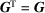.

#### Example

Let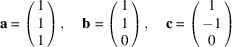be the basis of a lattice. Then the metric tensor of(with respect to the given basis) is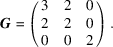With the help of the metric tensor the scalar products of arbitrary vectors, given as linear combinations of the lattice basis, can be computed from their coordinate columns as follows: If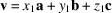and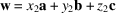, then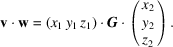From this it follows how the metric tensor transforms under a basis transformation. If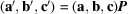, then the metric tensor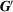ofwith respect to the new basisis given by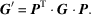An alternative way to specify the geometry of a lattice in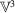is using the cell parameters, which are the lengths of the lattice basis vectors and the angles between them.

#### Definition

For a latticeinwith lattice basisthe cell parameters (also called lattice parameters, lattice constants or metric parameters) are given by the lengths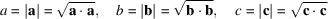of the basis vectors and by the interaxial angles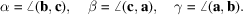Owing to the relation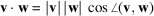for the scalar product of two vectors, one can immediately write down the metric tensor in terms of the cell parameters: